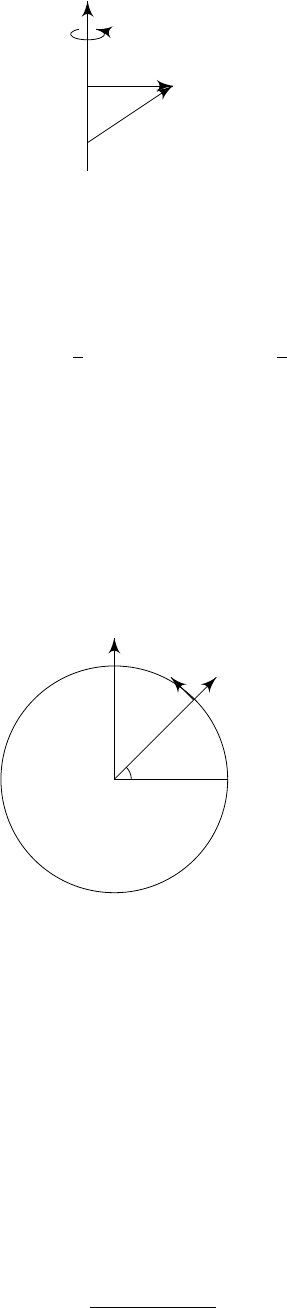5Rotating frames

IA Dynamics and Relativity5.2 The centrifugal force
What exactly does the centrifugal force do? Let ω = ω
ˆ
ω, where |
ˆ
ω| = 1. Then
mω × (ω × r) = m
(ω · r)ω (ω · ω)r
=
2
r
,
where
r
=
r
(
r·
ˆ
ω
)
ˆ
ω
is the projection of the position on the plane perpendicular
to
ω
. So the centrifugal force is directed away from the axis of rotation, and its
magnitude is
2
times the distance form the axis.
ω
r
r
Note that
r
· r
= r · r (r ·
ˆ
ω)
2
(|r
|
2
) = 2r 2(r ·
ˆ
ω)
ˆ
ω = 2r
.
So
mω × (ω × r) = −∇
1
2
2
|r
|
2
= −∇
1
2
m|ω × r|
2
.
Thus the centrifugal force is a conservative (fictitious) force.
On a rotating planet, the gravitational and centrifugal forces per unit mass
combine to make the effective gravity,
g
eff
= g + ω
2
r
.
This gravity will not be vertically downwards. Consider a point
P
at latitude
λ
on the surface of a spherical planet of radius R.
We construct orthogonal axes:
O
ω
P
ˆ
z
ˆ
y
λ
with
ˆ
x into the page. So
ˆ
z is up,
ˆ
y is North, and
ˆ
x is East.
At P , we have
r = R
ˆ
z
g = g
ˆ
z
ω = ω(cos λ
ˆ
y + sin λ
ˆ
z)
So
g
eff
= g + ω
2
r
= g
ˆ
z + ω
2
R cos λ(cos λ
ˆ
z sin λ
ˆ
y)
= ω
2
R cos λ sin λ
ˆ
y (g ω
2
R cos
2
λ)
ˆ
z.
So the angle α between g and g
eff
is given by
tan α =
ω
2
R cos λ sin λ
g ω
2
R cos
2
λ
.
This is 0 at the equator and the poles, and greatest when you are halfway
between. However, this is still tiny on Earth, and does not affect our daily life.